# tp框架实现数组翻页

``````//数组分页
function array_page(\$array,\$rows){
import("ORG.Util.Page"); //导入分页类
\$count=count(\$array);
\$Page=new Page(\$count,\$rows);
\$list=array_slice(\$array,\$Page->firstRow,\$Page->listRows);
\$data['list']=\$list;
\$data['page']=\$Page->show();
return \$data;
}
``````

##### 热门推荐
•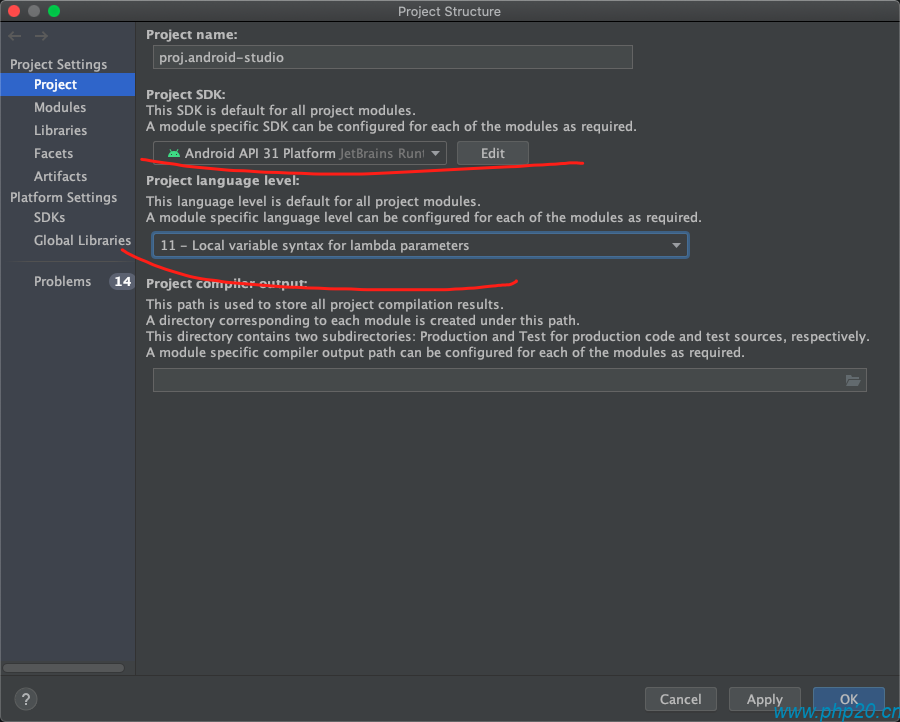浏览(2,407)
•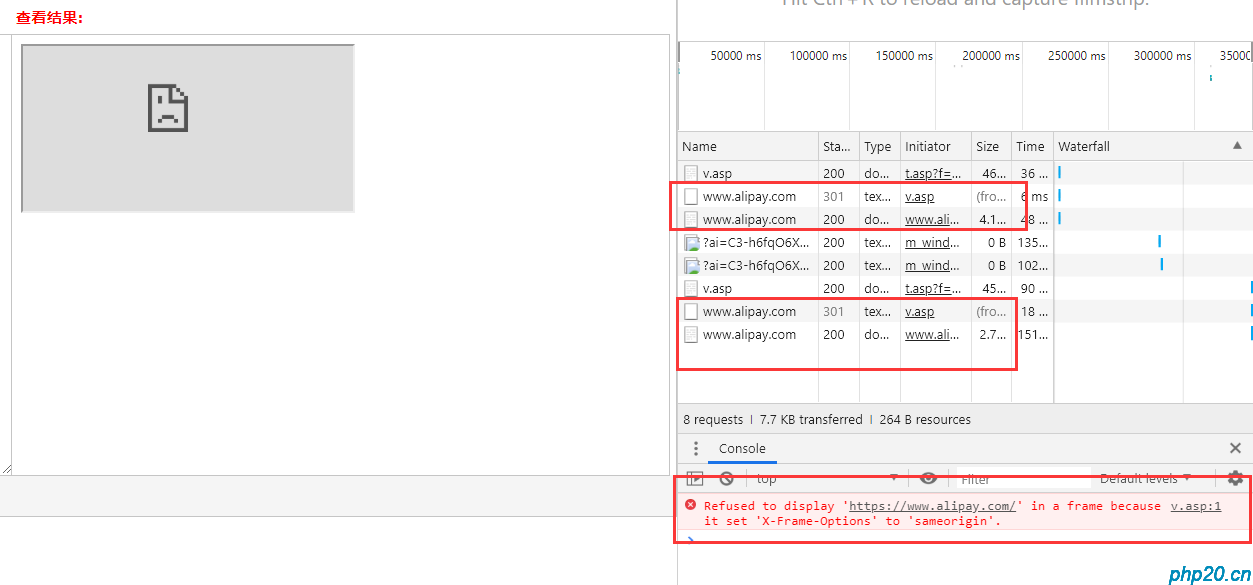浏览(1,735)
••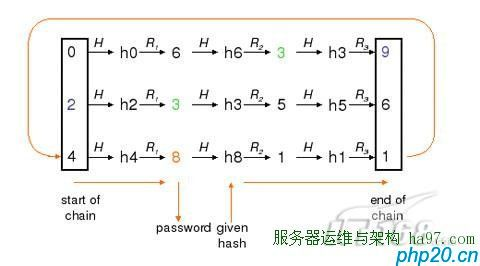•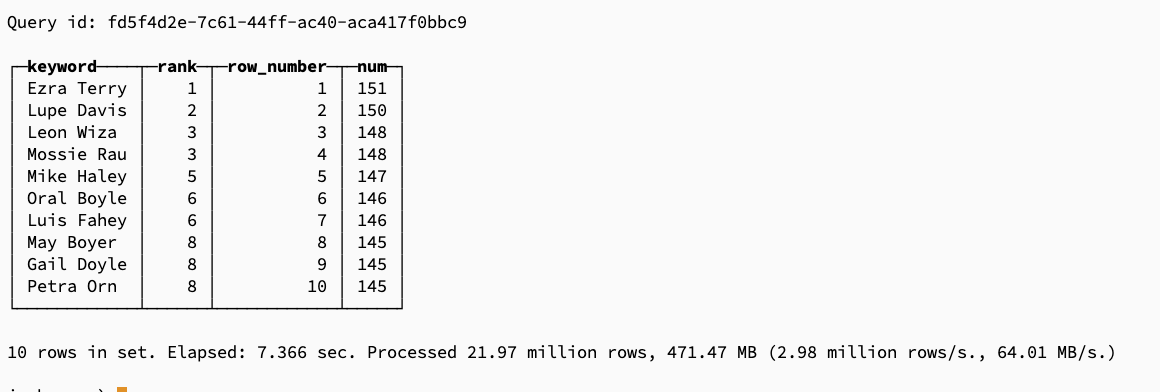浏览(1,232)
•浏览(1,177)
•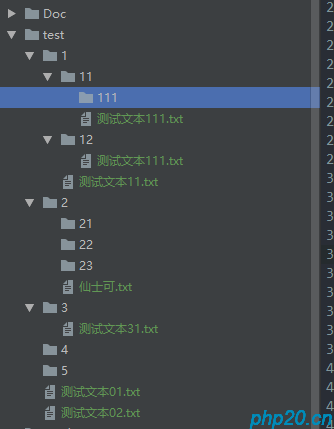浏览(1,160)
•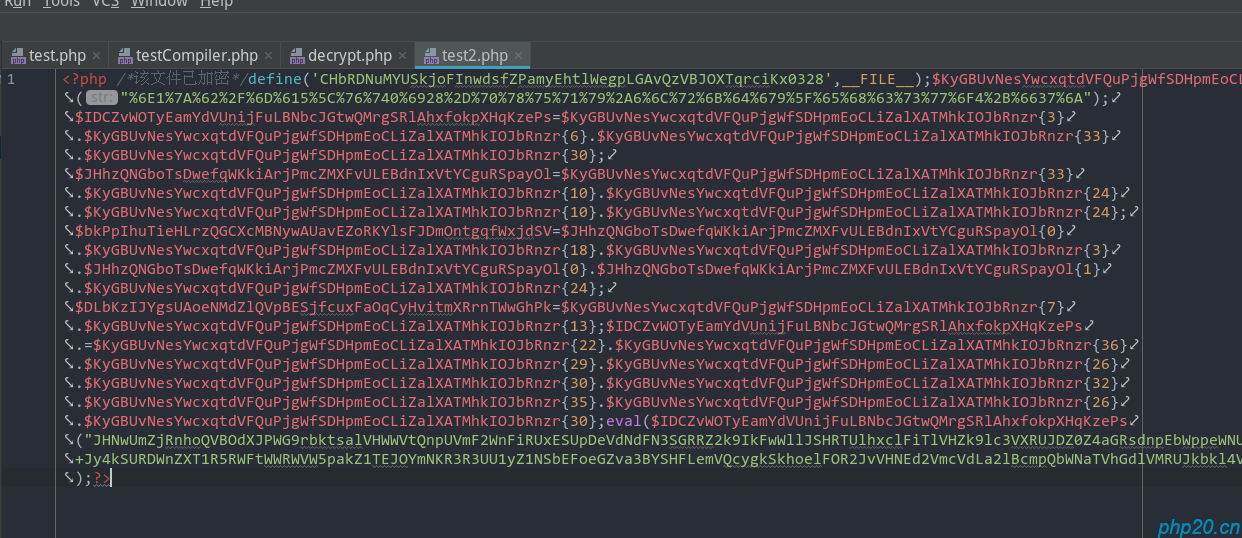浏览(1,150)
•浏览(1,096)
•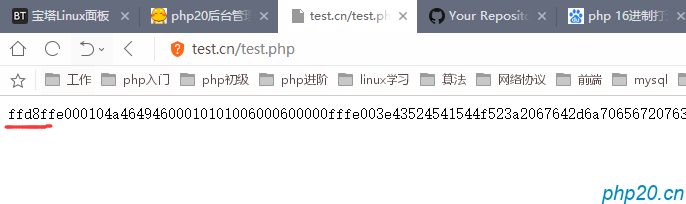浏览(1,070)